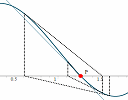Archive for July, 2015

IntMath Newsletter: Newton’s Method, Desmos activities

1. New interactive graph: Newton's Method
2. Newton's Method, accuracy and floating point numbers
3. Resource: Desmos Activities
4. Math puzzles
5. Math movie: Talks for people who hated math in high school
6. Final thought: Success often means failure first

Newton’s Method, accuracy and floating point numbersYouTube engineers faced a problem as the number of views for Gangnam Style approached 2 billion. I found related issues when developing the Newton's Method graph.

Newton’s Method interactive graphI added a new interactive graph that helps explain how Newton's Method works. Newton's Method uses differentiation to solve non-linear equations and is especially useful when we can't factor the equation. It's very commonly used by computers when solving math-based problems.

Full archive

See the blog sitemap for all previous articles.